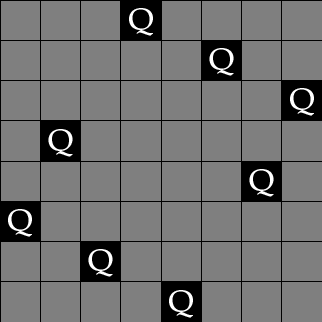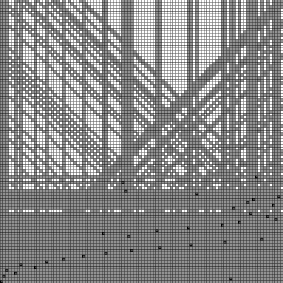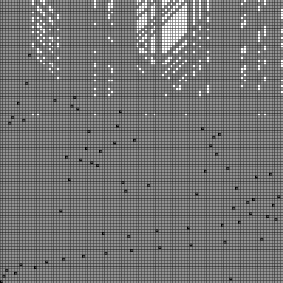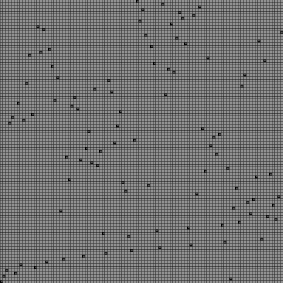Solving N-queens with Prolog

The task is to place N queens on an N×N chessboard in such a way that none of the queens is under attack.

Prolog Solution

In the programming language Prolog, it is easy to describe solutions of this task using CLP(FD) constraints which are commonly used for combinatorial tasks:

 ```n_queens(N, Qs) :- length(Qs, N), Qs ins 1..N, safe_queens(Qs). safe_queens([]). safe_queens([Q|Qs]) :- safe_queens(Qs, Q, 1), safe_queens(Qs). safe_queens([], _, _). safe_queens([Q|Qs], Q0, D0) :- Q0 #\= Q, abs(Q0 - Q) #\= D0, D1 #= D0 + 1, safe_queens(Qs, Q0, D1). ```

Full code: queens.pl

You can use SWI-Prolog > 5.6.50 to try it. Start it with:
```      \$ swipl queens.pl
```
You can then run queries like:
```      ?- N = 8, n_queens(N, Qs), labeling([ff], Qs).
Qs = [1, 5, 8, 6, 3, 7, 2, 4] .

?- N = 20, n_queens(N, Qs), labeling([ff], Qs).
Qs = [1, 3, 5, 14, 17, 4, 16, 7, 12, 18, 15, 19, 6, 10, 20, 11, 8, 2, 13, 9] .

?- N = 100, time((n_queens(N,Qs),labeling([ff],Qs))).
% 2,304,144 inferences, 0.553 CPU in 0.555 seconds (100% CPU, 4168963 Lips)
Qs = [1, 3, 5, 57, 59, 4, 64, 7, 58|...] .
```
The n-th integer in the list denotes the row of the queen that is placed in column n.

Animation

If you have the PostScript viewer "gs" installed, you can view an animation of the constraint solving process.

Here are a few example queries that you can try:
```      ?- show(4, [], Qs).

?- show(N, [], Qs).

?- show(50, [ff], Qs).
```
The arguments of the predicate show/3 are:
1. the number of queens
2. a list of labeling options as described in the CLP(FD) documentation
3. a list of row numbers, one for each column, such that the queens in these positions do not attack each other.
As a side-effect, you see an animation of the constraint solving process.

Sample PostScript file, a self-contained saved animation for 50 queens (open it with "gv" or "gs" to view it): queens50.ps

Sample animation (requires JavaScript), showing 80 queens with labeling strategy ff: queens80.

Here is a picture of a finished animation:
```      ?- show(8, [ff], Qs).
Qs = [1, 5, 8, 6, 3, 7, 2, 4] ;
Qs = [1, 6, 8, 3, 7, 4, 2, 5] ;
...
Qs = [3, 5, 2, 8, 1, 7, 4, 6] .
```Example with 100 queens:
```      ?- show(100, [ff], Qs).
Qs = [1, 3, 5, 57, 59, 4, 64, 7, 58|...] .
```Challenges: Find solutions for many queens. Find good labeling and allocation strategies. ...

Further reading: Neumerkel at al., Visualizing Solutions with Viewers.

More CLP(FD) examples: https://github.com/triska/clpfd

More about Prolog: The Power of Prolog

Main page